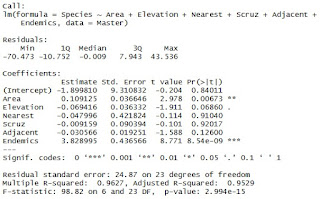## Pages

###R Tutorial 14.0

I have been a "Jabra Fan"(die hard fan) of Linear Regression, ever since I have started working in analytics space. The breadth and depth of OLS have been covered already in various of our blogs. In this blog we would understand "How to perform Linear Regression in R"

It is always a good feeling to write on your favorite topic !

Few or the previous blogs on Linear Regression :

It is time to learn linear regression in R.

# Let's first install few packages that would be used in the course of regression

install.packages("car")
install.packages("caret")
install.packages("ggplot2")

# Let's now call the packages

library(ggplot2)
library(car)
library(caret)

# We have chosen data gala (in faraway library) for demonstrating Linear Regression in R

install.packages("faraway")
library(faraway)

Master =  gala

# You now have a data Master in Environment with 7 variables, and 30 observation
#Following are the variable in data
#The dataset contains the following variables
#Species - the number of plant species found on the island
#Endemics - the number of endemic species Area the area of the island (sq.km.)
#Elevation - the highest elevation of the island (m)
#Nearest - the distance from the nearest island (km)
#Scruz - the distance from Santa Cruz island (km)

# The objective of linear regression here would be to establish a relationship of Species over other variables
# In technical terms, Species would be Y variable (Dependent one) and other would serve as X variables (Independent variables).

# Let's first understand the correlation among the variables.

attach(Master)
require(Hmisc)
rcorr(as.matrix(Master),type ="pearson")

# Seems like Species is most correlated with Endemics, Elevation and Area,
# I don't know much about botany to comment on this, so let's focus on coding part

# Let's now do a driver Analysis in order to see any need of transformation in order to satisfy the assumption of linearity - for continuous data onlyclick to enlarge
attach(Master)
layout(matrix(c(1,2,3,4,5,6),2,3))
plot(Species, Endemics)
plot(Species, Area)
plot(Species, Elevation)
plot(Species, Nearest)
plot(Species, Scruz)

# These plot can be used for identifying outliers (in univariate sense only) and also to see if any transformation is required.

# Outliers can be capped, you should use your own business acumen to do such capping in your project.
# Here for example we are doing following capping:

Master\$Elevation =  ifelse(Elevation > 1000 ,1000,Elevation)
Master\$Area =  ifelse(Area > 1000 ,1000,Area)

# For sake of simplicity, I am not delving into this part much

library(Hmisc)
rcorr(as.matrix(Master))
library(corrgram)
corrgram(Master, order=F, lower.panel=panel.pie,
upper.panel=panel.ellipse, text.panel=panel.txt,
main="Species on Isalands")

# Let's  go ahead just like that and run first linear regression model
# Do attach the data again as you might have performed transformation and capping aboveclick to enlarge

attach(Master)
model_1 = lm(Species ~ Area + Elevation + Nearest + Scruz + Adjacent+ Endemics,
data = Master)
summary(model_1)

# Syntax is very simple
# model = lm(y~x1+x2+x3 ....., data = dataset_that_you_want_to_work_with)

# Based on variance inflation stats (vif), you can drop variables ... all of this have been explained in our previous blogs on linear regression.

# Auto selection of variables - MASS package is required for that
# it exploits pre-built basic model

install.packages("MASS")
library(MASS)
step <- stepAIC(model_1, direction="both")
summary(step)

# thus we got the stepwise selection of the variables,
# you can also use "backward" or "forward" to get backward or forward selection of variables.

# Suppose we finally use our business acumen and decide upon final set of variables and run the model

model_2 <- lm(Species ~ Endemics+Area+Elevation   , data = Master)
summary(model_2)

# Let's now store the predicted value
y_hat = fitted(model_2)
result = cbind(Master,y_hat)

And we are done with the model... are we really done?

No, we still need to check certain assumptions such as homoscedasticity, independence and normality of error term.

We will cover these things in an upcoming blog, till then ...

Enjoy reading our other articles and stay tuned with us.

Kindly do provide your feedback in the 'Comments' Section and share as much as possible.